Descriptive Statistics

# 12 Skewness and the Mean, Median, and Mode

Consider the following data set.
4; 5; 6; 6; 6; 7; 7; 7; 7; 7; 7; 8; 8; 8; 9; 10

This data set can be represented by following histogram. Each interval has width one, and each value is located in the middle of an interval.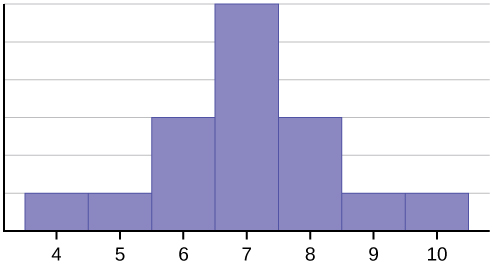The histogram displays a symmetrical distribution of data. A distribution is symmetrical if a vertical line can be drawn at some point in the histogram such that the shape to the left and the right of the vertical line are mirror images of each other. The mean, the median, and the mode are each seven for these data. In a perfectly symmetrical distribution, the mean and the median are the same. This example has one mode (unimodal), and the mode is the same as the mean and median. In a symmetrical distribution that has two modes (bimodal), the two modes would be different from the mean and median.

The histogram for the data: 4566677778 is not symmetrical. The right-hand side seems “chopped off” compared to the left side. A distribution of this type is called skewed to the left because it is pulled out to the left. We can formally measure the skewness of a distribution just as we can mathematically measure the center weight of the data or its general “speadness”. The mathematical formula for skewness is: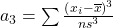. The greater the deviation from zero indicates a greater degree of skewness. If the skewness is negative then the distribution is skewed left as in (Figure). A positive measure of skewness indicates right skewness such as (Figure).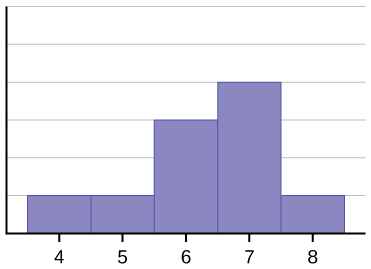The mean is 6.3, the median is 6.5, and the mode is seven. Notice that the mean is less than the median, and they are both less than the mode. The mean and the median both reflect the skewing, but the mean reflects it more so.

The histogram for the data: 67777888910, is also not symmetrical. It is skewed to the right.The mean is 7.7, the median is 7.5, and the mode is seven. Of the three statistics, the mean is the largest, while the mode is the smallest. Again, the mean reflects the skewing the most.

To summarize, generally if the distribution of data is skewed to the left, the mean is less than the median, which is often less than the mode. If the distribution of data is skewed to the right, the mode is often less than the median, which is less than the mean.

As with the mean, median and mode, and as we will see shortly, the variance, there are mathematical formulas that give us precise measures of these characteristics of the distribution of the data. Again looking at the formula for skewness we see that this is a relationship between the mean of the data and the individual observations cubed.where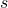is the sample standard deviation of the data,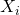, and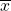is the arithmetic mean and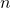is the sample size.

Formally the arithmetic mean is known as the first moment of the distribution. The second moment we will see is the variance, and skewness is the third moment. The variance measures the squared differences of the data from the mean and skewness measures the cubed differences of the data from the mean. While a variance can never be a negative number, the measure of skewness can and this is how we determine if the data are skewed right of left. The skewness for a normal distribution is zero, and any symmetric data should have skewness near zero. Negative values for the skewness indicate data that are skewed left and positive values for the skewness indicate data that are skewed right. By skewed left, we mean that the left tail is long relative to the right tail. Similarly, skewed right means that the right tail is long relative to the left tail. The skewness characterizes the degree of asymmetry of a distribution around its mean. While the mean and standard deviation are dimensional quantities (this is why we will take the square root of the variance ) that is, have the same units as the measured quantities, the skewness is conventionally defined in such a way as to make it nondimensional. It is a pure number that characterizes only the shape of the distribution. A positive value of skewness signifies a distribution with an asymmetric tail extending out towards more positive X and a negative value signifies a distribution whose tail extends out towards more negative X. A zero measure of skewness will indicate a symmetrical distribution.

Skewness and symmetry become important when we discuss probability distributions in later chapters.

### Chapter Review

Looking at the distribution of data can reveal a lot about the relationship between the mean, the median, and the mode. There are three types of distributions. A right (or positive) skewed distribution has a shape like (Figure). A left (or negative) skewed distribution has a shape like (Figure). A symmetrical distrubtion looks like (Figure).

### Formula Review

Formula for skewness:Formula for Coefficient of Variation: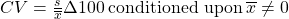Use the following information to answer the next three exercises: State whether the data are symmetrical, skewed to the left, or skewed to the right.

11122223333333344455

The data are symmetrical. The median is 3 and the mean is 2.85. They are close, and the mode lies close to the middle of the data, so the data are symmetrical.

161719222222222223

87878787878889899091

The data are skewed right. The median is 87.5 and the mean is 88.2. Even though they are close, the mode lies to the left of the middle of the data, and there are many more instances of 87 than any other number, so the data are skewed right.

When the data are skewed left, what is the typical relationship between the mean and median?

When the data are symmetrical, what is the typical relationship between the mean and median?

When the data are symmetrical, the mean and median are close or the same.

What word describes a distribution that has two modes?

Describe the shape of this distribution.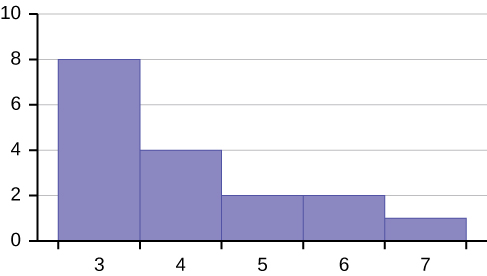The distribution is skewed right because it looks pulled out to the right.

Describe the relationship between the mode and the median of this distribution.Describe the relationship between the mean and the median of this distribution.The mean is 4.1 and is slightly greater than the median, which is four.

Describe the shape of this distribution.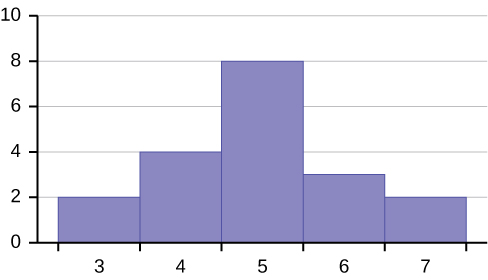Describe the relationship between the mode and the median of this distribution.The mode and the median are the same. In this case, they are both five.

Are the mean and the median the exact same in this distribution? Why or why not?Describe the shape of this distribution.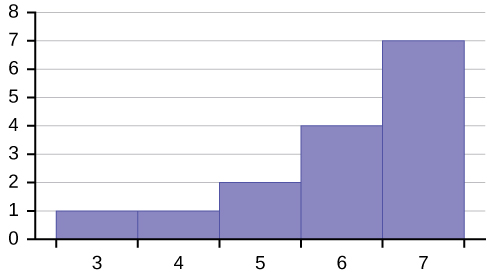The distribution is skewed left because it looks pulled out to the left.

Describe the relationship between the mode and the median of this distribution.Describe the relationship between the mean and the median of this distribution.The mean and the median are both six.

The mean and median for the data are the same.

345566667777777

Is the data perfectly symmetrical? Why or why not?

Which is the greatest, the mean, the mode, or the median of the data set?

111112121212131517222222

The mode is 12, the median is 12.5, and the mean is 15.1. The mean is the largest.

Which is the least, the mean, the mode, and the median of the data set?

5656565859606264646567

Of the three measures, which tends to reflect skewing the most, the mean, the mode, or the median? Why?

The mean tends to reflect skewing the most because it is affected the most by outliers.

In a perfectly symmetrical distribution, when would the mode be different from the mean and median?

### Homework

The median age of the U.S. population in 1980 was 30.0 years. In 1991, the median age was 33.1 years.

1. What does it mean for the median age to rise?
2. Give two reasons why the median age could rise.
3. For the median age to rise, is the actual number of children less in 1991 than it was in 1980? Why or why not?